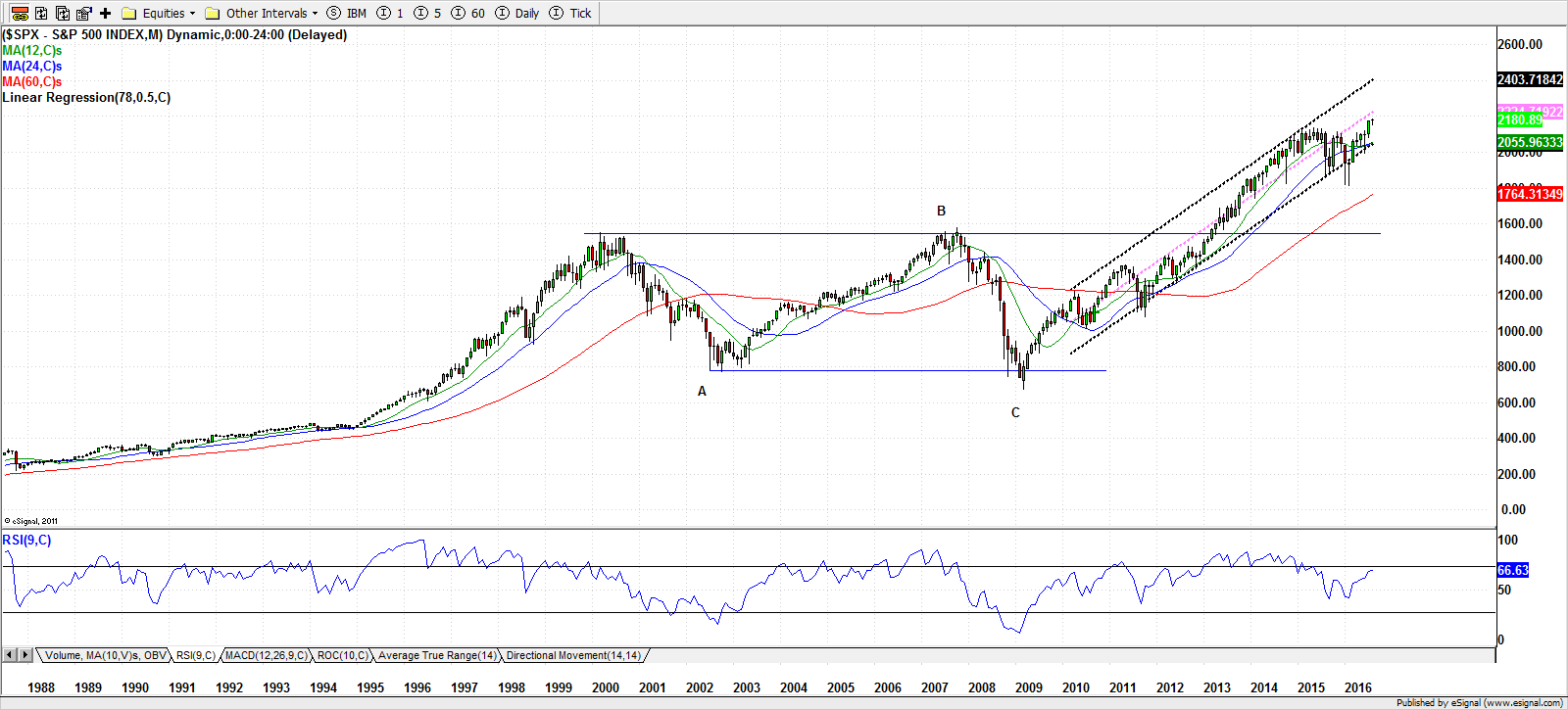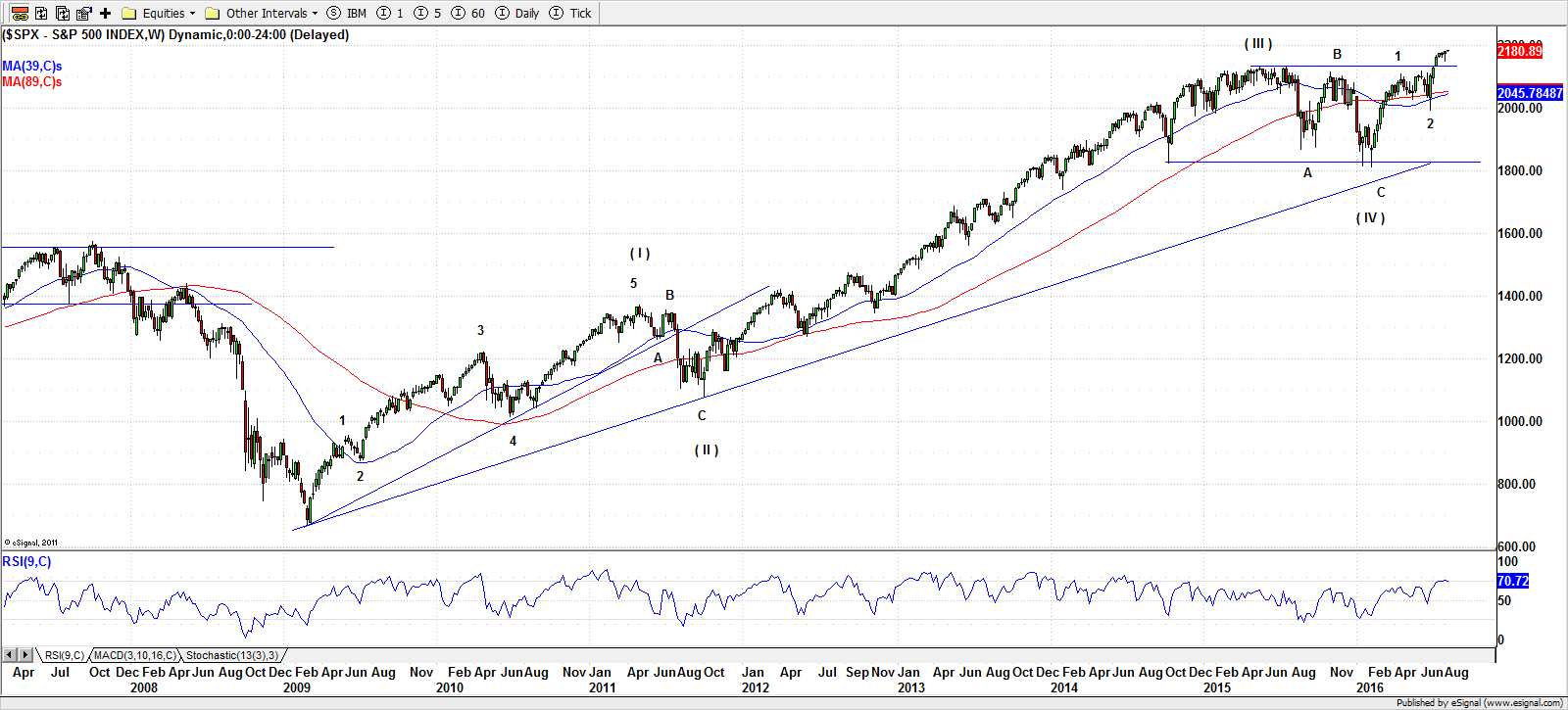# Here’s Looking At You, S&P 500

## Long Term Still Looking GreatOn the monthly-timeframe, S&P 500 is on an ever extending up move. In the last twenty years, markets had two major melt-downs and two major tops excluding the current rally. These reversal points formed a nice looking rectangle trading box for the S& 500. The upper limit is bounded by the highs of 1553.11 and 1576.09 reached in March 2000 and October 2007 respectively. The lower limit is bounded by the lows of 768.77 and 666.79 reached in October 2002 and March 2009. The index broke above this rectangle in March 2013. Since then it has been on an upward trend.

The height of the box is roughly 909 points (from highest to lowest). The measured target of this patterns using Fibonacci levels are (i) 2138.04 at 61.8% extension; (ii) 2485.39 at 100% extension; and (iii) 3047.34 at 161.8% extension

We can view this as an ABCD patterns too, whereas ‘A’ is 768.77, ‘B’ is 1576.09 and ‘C’ is 666.79. Using the Fibonacci extension of BC from point ‘C’, we get the projected levels of ‘D’ to be: (i) 2138.04 using 161.8% extension and (ii) 3047.34 using 261.8%

The 2015 high was 2134.72 reached in May, which corresponds with 61.8% extension of the rectangle trading box and 161.8% extension of the ABCD pattern. The index took more than thirteen months to break above this level. The next target in play is 3047.34, which is close to 40% above the current level. The annual rate if change for S&P 500 since 1971 has been approximately 7.13%. Using this average, it will take S&P 500 five years to reach 3047.34.

The two major downturns in the index also coincided with the cross-over of 12-months and 24-months moving averages. In April 2001 and July 2008, the 12-month MA crossed below 24-month MA. Both averages and the price was already falling before this cross over.

In May 2016, the 12-month MA crossed below 24-month MA. In July it again crossed above the slow MA. However, the price and the 24-month MA were rising when the first cross over occurred.

Also, in 2000 and 2007, the 9-period RSI was above 70 and making a diverging from the price. So far this time the RSI has not crossed above the overbought levels.

## Weekly Corroborates Monthly

After making the bottom in March 2009, S&P 500 has been on a uptrend. We can use Elliott Wave to analyze this move. Our assessment, using Elliott Wave rules and guidelines, is that we just completed wave 4 of the wave structure. Mind you Elliott Waves are of various degrees and one wave may contain various waves of smaller degree. The wave structure that we have identified is of long-term duration.The start of the wave sequence is from the low of 666.79 reached in the week of March 2, 2009. The wave ( I ) ended 1370.58 during the week of May 2, 2011. This wave included a smaller wave structure denoted by numerals 1, 2, 3, 4, and 5. In this motive wave, the wave 3 is an extension.

The wave 5 is followed by a corrective wave denoted by A, B and C. The wave C also indicates the end of wave ( II ). This was the low of 1074.77 reached during the week of October 3, 2011.

The wave ( III ) is an extension wave. This wave too contain other motive wave sequence of smaller degrees. For brevity, and to avoid chart-cluttering, we did not mark them. The wave ended at 2134.72 during the week of May 18, 2015.

This was followed by a corrective wave A, B and C, which had a structure of flat. This corrective wave ended with wave ( IV ) at 1810.10 during the week of February 8, 2016.

Since then another wave sequence of smaller degree has started. The wave 1 of this lesser degree motive waves ended 2111.05 during the week of April 18, 2016. The wave 2 ended at 1991.68 during June 27, 2016.

Using the guidelines for measurements we can establish some targets for the wave ( V ) of the higher degree motive wave.

Using the guideline that if one of the motive waves is an extension then the other two are either of same magnitude and duration or one of them is 61.8% of other. Wave ( I ) lasted for 113 weeks and gained 703.79 points. This gives us a target of 2513.89 and an estimated date in April 2018. This is 15% above the current levels. Using 61.8%, we get a target of 2245.05 by May 2017. This is 2.9% above current level.

The other scenario is when the wave ( I ) is 61.8% of the wave ( I V ). This gives us a target of 2948.92 in May 2019.

We can also estimate the wave ( V ) using the emerging smaller degree motive wave since wave ( IV ). The wave 1 ended at 2111.05. Since wave 3 can not be smaller than wave 2, it needs to end at least beyond 2292.63, which in turn means that the wave ( V ) needs to go beyond that level.